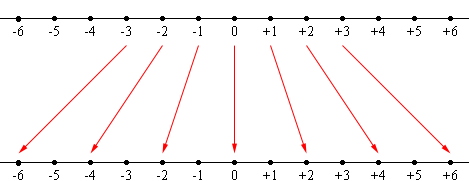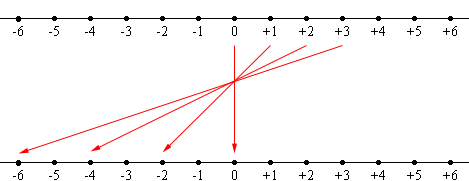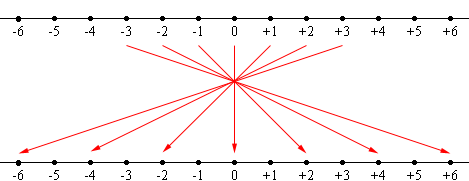SEARCH HOMEMath Central Quandaries & QueriesQuestion from stephanie, a student: hi, I'm currently working in a grade 8 class where the students are learning about integers. the students were asked to find examples of how integers are applied in real life. they were able to find applications for: adding and subtracting both positive and negative integers. however, we could not find an example of where two negative integers would be either divided or multiplied together in real life. For example: (-2)(-4)= +8. please help.Hi Stephanie,

This is a natural question for a student to ask and one for which I am not sure I have a very satisfactory answer. My answer as a mathematician is that (-2)(-4) = 8 is a consequence of the distributive law. The distributive law is so useful and it adds so much to the beauty and structure of arithmetic that I want it to be true in every case and hence (-2)(-4) = 8.

This is not a very satisfactory response and doesn't address your request for an example in "real life". (I really dislike this term! As a teacher you will most likely be doing mathematics every day of your life and I expect your life is as real as anyone else's. I have started using the term "math beyond school".) As I see it, the question amounts to "is there a use of arithmetic and an interpretation of multiplication where it is natural to multiply a negative number by a negative number?"

In many of the situations where we see negative numbers the concept of multiplication of two negatives is not natural. I think of temperature, my savings account where a deposit is positive and a withdrawal is negative, height above sea level and so on. I want to show you an interpretation of multiplication where the multiplication of two negative numbers is natural.

I am thinking of the number line and multiplication is an action, for example doubling or tripling. Multiplying by 2 stretches the line by a factor of 2.Multiplication by 2

Likewise multiplication by 3 is the action that stretches the line by a factor of 3. You can even extend this to fractions where multiplication by a fraction less than 1 is a contraction of the line.

What about multiplication by -2? We know that -2 × 0 = 0, -2 × 1 = -2, -2 × 2 = -4, -2 × 3 = -6 so there is again a stretching by a factor of 2 but the direction of the line is reversed.Multiplication by -2

Under this action of stretching by 2 and reversing the direction what happens to the negative numbers?Multiplication by -2

-2 × -1 = +2, -2 × -2 = +4, -2 × -3 = +6 and so on.

This interpretation of multiplication might not seem particularly applied or natural, but is is. It supplies the foundation for building a mathematical model of the motion of an object along a line or even the motion of an object in 3-space.

This example comes from Barry Mazur's book Imagining Numbers (particularly the square root of minus fifteen).

I hope this helps,
HarleyMath Central is supported by the University of Regina and The Pacific Institute for the Mathematical Sciences.